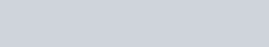## Nonlinear Stresses from Linear FEA

Learn how a Nastran linear-elastic analysis can be corrected to take into account nonlinear material behavior. Take advantage of the benefits of a linear analysis without sacrificing precision.

Challenges:

• Time limitations
• Model size and computational resources
• Large number of load cases

Values:

• Capture nonlinear behavior using linear FEA results
• Quick to run and iterate on
• Easily incorporate optimization into the design process
• Can create scripts to programmatically compute nonlinear stresses

## Linear-Elastic Analysis

In performing structural analysis, it is always preferable to start simple and add complexity and detail as needed. As an example, when performing a static structural analysis, the starting point should be a linear solution (SOL 101).

One common simplification is to use a linear material model. A linear-elastic analysis constrains the finite element solution to the linear portion of a material’s stress-strain curve. This region is shown below. In this region, the material is accurately characterized by the relationship σ = Eε.However, reality is highly non-linear. Designing structures to be within the linear-elastic region of their stress-strain curve would significantly limit the design space. What happens when the stress in a component is outside of this linear region? Commonly local stress concentrations result in small regions in a structure having large stresses. Should these local regions result in redesign or added material (weight)?

To take into account this nonlinear region, a nonlinear finite element analysis could be set up and run. In Simcenter 3D this is very easy to do. However, caution must be exercised. In certain instances, running a non-linear solution is both unnecessary and prohibitively expensive (in terms of engineering and computational time). What can be done in these cases? That is where the Neuber plasticity correction comes in. In the example below, the process for determining the nonlinear stress from a linear FEA analysis will be presented. Note that the Neuber plasticity correction applies to regions of local stress concentration. This method is not intended for gross plasticity (plastic bending, etc.).

## Step 1: Build a Finite Element Model

A simple geometry has been created in NX. This geometry was then used to construct a finite element model. The key dimensions of the part are shown below.An axial load of 8,000 lbf was then applied to the model and a linear-elastic solution was run using Simcenter Nastran (formerly known as NX Nastran).  Note that M4 Engineering is an officially licensed reseller of Simcenter Nastran.

## Step 2: Review the Linear-Elastic Result

Once the linear-elastic model has been run, the component stresses can be checked. In this case, the geometry is simple and enables comparison with standard hand calculation methods.

Peterson’s Stress Concentration Factors was used for this purpose. The peak stress at the hole was determined from the following chart:With d/H equal to 0.25, Ktg is found to be 3.24. The maximum stress can then be found by multiplying the nominal stress away from the bolt hole by this stress concentration factor. The nominal stress is found to be:The max principal stress computed using the finite element model is 51,179 psi.This is within two percent of the hand calculation. The von Mises stress computed by the finite element model is 52,342 psi and is shown below.Note that for the 6061-T6 material that was chosen for this plate, 52 ksi is well above both the yield and ultimate strength of the material, 41.0 ksi and 42.5 ksi respectively . Further analysis is required.

## Step 3: Correct Linear-Elastic Stress

The simplest approach to determine whether or not this linear-elastic stress is an issue would be to perform a hand calculation using the Neuber plasticity correction. This method is both accurate and quick. The Neuber correction allows determination of total strain (elastic + plastic) and nonlinear stress using a hand analysis method. This method is especially helpful during the initial design / optimization phase of product development. Instead of iterating multiple times to solve a single load case, multiple designs and load cases can be considered. This can cut a significant portion of development time out of the design cycle!

To perform the Neuber correction, the following must be used:In other words, the product of the linear-elastic stress and strain remains constant. As such, a point on the material stress strain curve that meets this requirement can be found.

The nonlinear stress at different strain levels can be determined while holding the above product constant. These stress-strain sets will result in the dotted blue line shown in the figure below. The intersection of the dotted blue line with the stress-strain curve is the nonlinear stress state.The nonlinear stress computed using this approach is 41.489 ksi. This is slightly above the 0.2% offset yield strength of the material but is significantly less alarming than 52 ksi.

## Step 4: Compare Result to Nonlinear Analysis

The same example problem was run using a nonlinear material model in Nastran solution 106. The nonlinear von Mises stress from this analysis is presented below:The peak stress is 40.735 ksi. The stress computed using the Neuber plasticity correction is within 2% of this result. Depending on the model size and number of load cases, the full-fledged nonlinear solution could have taken days to run. This correction took 15 minutes.

## Summary

The rule of thumb in stress analysis is start simple and add complexity. A linear-elastic model is often sufficient for many problems. When a linear-elastic model stress exceeds the linear portion of the stress-strain curve at a local stress concentration, the Neuber plasticity correction can be used to determine the effect of material nonlinearity. This method is preferable to a full-fledged nonlinear analysis in many instances, such as optimization, large model sizes, and large numbers of load cases.

##Written by Vitaliy Rezekulov

Vitaliy Rezekulov is a Senior Engineer with a background in structural analysis. He has worked on some interesting linear and nonlinear problems. Outside of work he enjoys playing board games and soccer with friends.